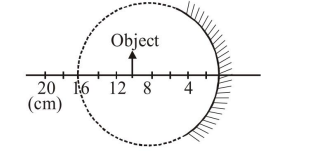# Solve this following

Question:A spherical mirror is obtained as shown in the figure from a hollow glass sphere. If an object is positioned in front of the mirror, what will be the nature and magnification of the image of the object ? (Figure drawn as schematic and not to scale)

1. Inverted, real and magnified

2. Erect, virtual and magnified

3. Erect, virtual and unmagnified

4. Inverted, real and unmagnified

Correct Option: 1

Solution:

$\mathrm{f}=\frac{-8}{2}=-4 \mathrm{~cm}$

$\mathrm{u}=-10 \mathrm{~cm}$

$\mathrm{v}=?$

as $\frac{1}{v}+\frac{1}{u}=\frac{1}{f}$

$\frac{1}{v}+\left(\frac{1}{-10}\right)=\frac{1}{-4}$

$\frac{1}{v}=\frac{1}{10}-\frac{1}{4}$

$\frac{1}{v}=\frac{4-10}{40}$

$v=\frac{40}{-6}$

$v=\frac{-20}{3}$

$\mathrm{m}=\frac{-\mathrm{v}}{\mathrm{u}}$

$\mathrm{m}=\frac{-\left(-\frac{20}{3}\right)}{-10} \Rightarrow \mathrm{m}=\frac{-2}{3}$

or image will be real, inverted and unmagnified.